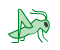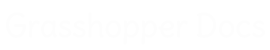﻿ Twisted Box Through Curves - Pufferfish - Component for Grasshopper | Grasshopper DocsCommunity documentation for Grasshopper add-ons & pluginsColor
 Curve
 Domain
 List
 Mesh
 Number
 Plane
 Point
 SubD
 Surface
 Transform
 Twisted Box
 Vector

# Twisted Box Through Curves

## Inputs

NameIDDescriptionType
CurvesCSet of curves to create twisted box array throughCurve
ThicknessTOverall thickness of the twisted box array, offset from the curvesNumber
Parameters UuDivision parameters through curves {u} domain ({u}0.0 = Curve at C start, {u}1.0 = Curve at C end), unless Normalized is False For best results {u} parameters should be between 0 and 1, in numerical order, and non duplicateNumber
Parameters VvDivision parameters along curve {v} domains ({v}0.0 = Curve starts, {v}1.0 = Curve ends) For best results {v} parameters should be between 0 and 1, in numerical order, and non duplicateNumber
Parameters WwDivision parameters along thickness {w} domain ({w}0.0 = Curves, {w}1.0 = Thickness) For best results {w} parameters should be between 0 and 1, in numerical order, and non duplicateNumber
NormalizedNIf False, the rules for {u} parameters are ({u}0.0 = Curve at index 0, {u}Index number of last curve = Curve at last index) For best results {u} parameters should be between 0 and the index of the last curve, in numerical order, and non duplicateBoolean
EqualizedEIf True, parameters {u} distribution is evaluated along the interpolation path without considering the locations of the input curve setBoolean
WeightedWIf True, parameters {v} distribution is weighted by the input curves curvaturesBoolean
LoopbackLIf True, allow the parameters {u} interpolation path to continue from the end back to the start as a closed loop (if Normalized is False, increase the division parameters {u} range maximum by 1)Boolean
Interpolation TypeIDetermines how the {u} interpolation path is interpolated 0 = Straight 1 = Normal 2 = Tight 3 = UniformInteger
RefitROptional Refit match method. (No Integer or 0 = Off, Integer greater than 0 = On and curve degree of refit) If an integer greater than zero, Refit match method is used if possible. When input curves are refit their control points are redistributed, added to, and removed from based on the curves curvature and the input integer degree, while trying to maintain their shapes. Refit results in tighter shaped twisted boxes, with curvature based control point distribution.Integer
Point SamplePOptional Point Sample match method. (No Integer or 0 = Off, Integer greater than 0 = On and amount of sample points) If an integer greater than zero, Point Sample match method is used. When input curves are sampled their control points are recreated by equally dividing the curve by the input integer point count. Point Sample results in looser shaped twisted boxes, with uniform control point distribution.Integer

## Outputs

NameIDDescriptionType
Twisted BoxesTBResulting twisted box array through curvesTwisted Box

## Video Tutorials

Site design © Robin Rodricks.   Pufferfish and associated data © 2023 Michael Pryor.
Rhinoceros and Grasshopper are registered trademarks of Robert McNeel & Associates.  Hosted by GitHub